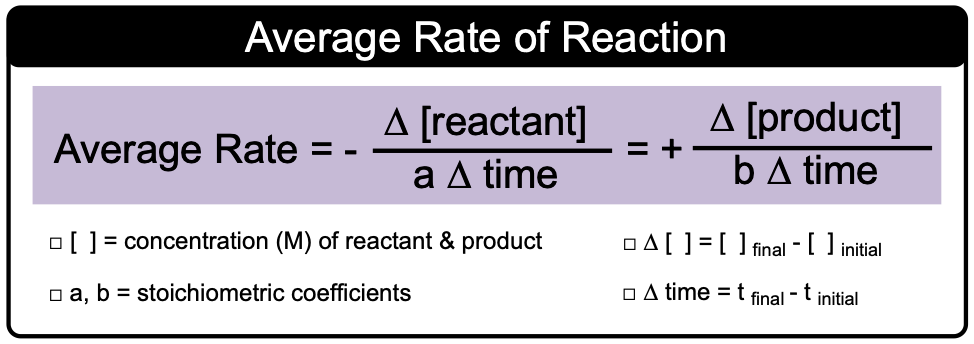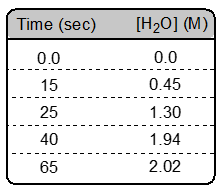Clutch Prep is now a part of Pearson
Ch.13 - Chemical KineticsWorksheetSee all chapters

# Average Rate of Reaction

See all sections
Sections
Intro to Chemical Kinetics
Energy Diagrams
Catalyst
Factors Influencing Rates
Average Rate of Reaction
Stoichiometric Rate Calculations
Instantaneous Rate
Collision Theory
Arrhenius Equation
Rate Law
Reaction Mechanism
Integrated Rate Law
Half-Life

Average (General) Rate is the change in the concentration of a compound over a period of time.

###### Average Rate

Concept #1: Average Rate of ReactionExample #1: Provide the expressions for rate of disappearance of reactants and rate of appearance of products for the following reaction: 2 C3H7OH (l) + 9 O2 (g) ⟶ 6 CO2 (g) + 8 H2O (g)

Example #2: Calculate the average rate of change in concentration of NO in the first 5 seconds of the reaction if the concentration dropped from 1.3 M to 1.09 M.

2 NO (g) + 2 H2 (g) ⟶ N2 (g) + 2 H2O (g)

Practice: Consider the following reaction: 2 H2O2 (aq) → 2 H2O (l) + O2 (g)

Calculate the rate of the reaction between 25 sec and 65 sec.